# Rational and Irrational Numbers Rational Number Rational Number

• Slides: 24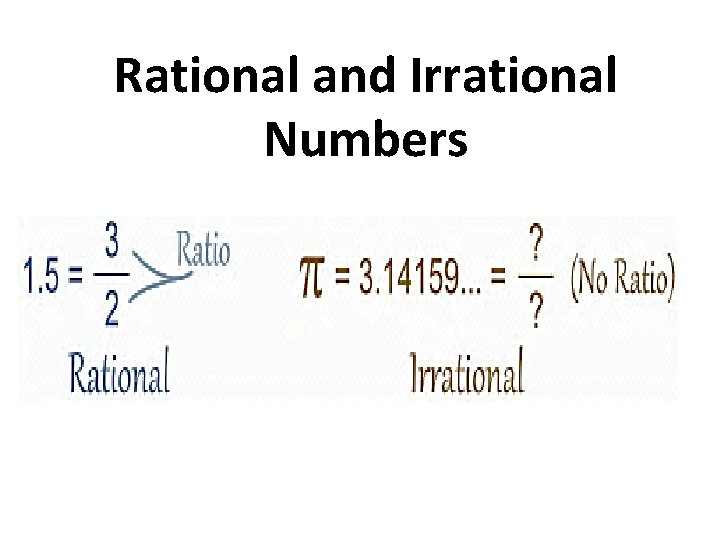Rational and Irrational Numbers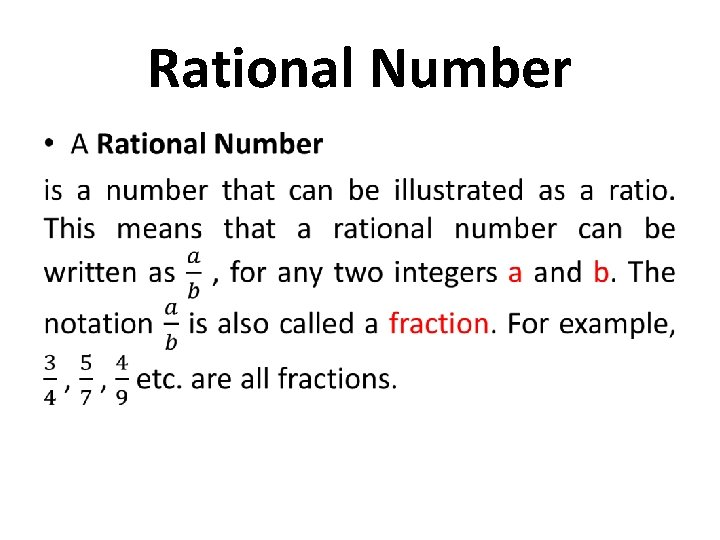Rational Number •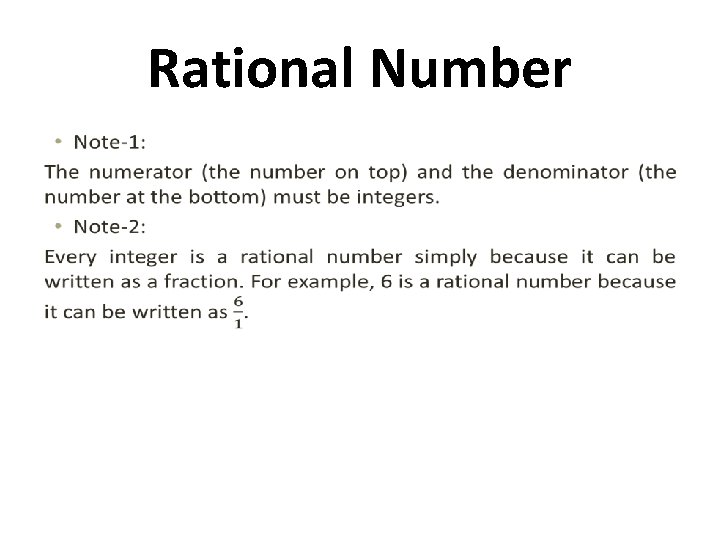Rational Number •Rational Number Rational numbers are numbers which are either repeated, or terminated. Like 0. 7645 and 0. 232323. . . are both rational numbers.Rational Number •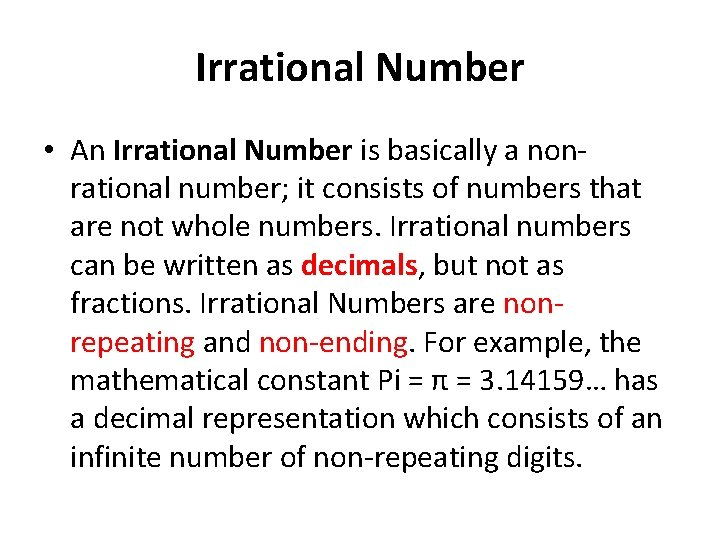Irrational Number • An Irrational Number is basically a nonrational number; it consists of numbers that are not whole numbers. Irrational numbers can be written as decimals, but not as fractions. Irrational Numbers are nonrepeating and non-ending. For example, the mathematical constant Pi = π = 3. 14159… has a decimal representation which consists of an infinite number of non-repeating digits.Irrational Number • The value of pi to 100 significant figures is 3. 14159265358979323846264338327950288 4197169399375105820974944592307816406 286208998628034825342117067. . . Note: Rational and Irrational numbers both exist on the number line.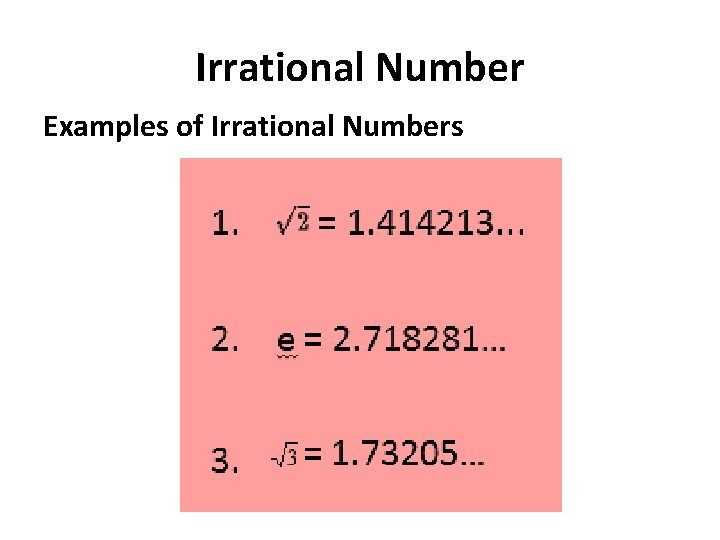Irrational Number Examples of Irrational Numbers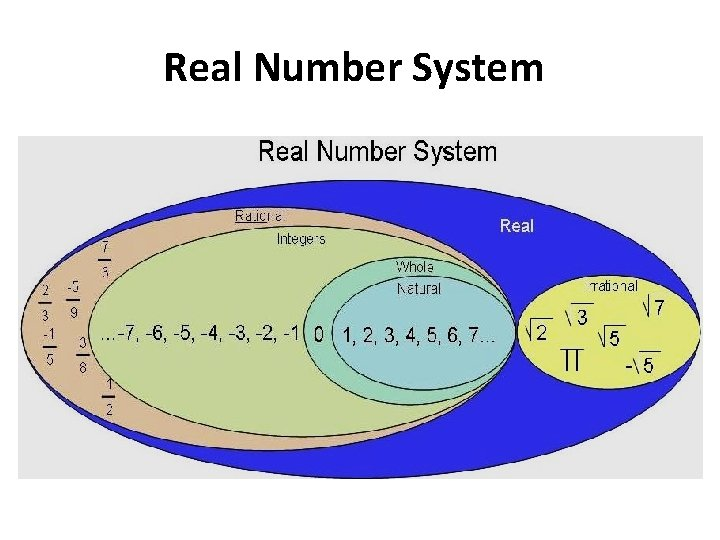Real Number SystemReal Number • Real numbers consist of all the rational and irrational numbers. • The real number system has many subsets: – Natural Numbers – Whole Numbers – Integers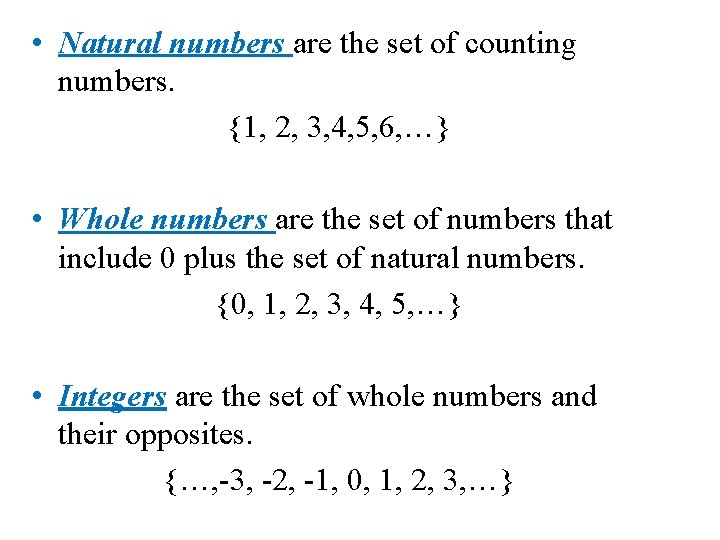• Natural numbers are the set of counting numbers. {1, 2, 3, 4, 5, 6, …} • Whole numbers are the set of numbers that include 0 plus the set of natural numbers. {0, 1, 2, 3, 4, 5, …} • Integers are the set of whole numbers and their opposites. {…, -3, -2, -1, 0, 1, 2, 3, …}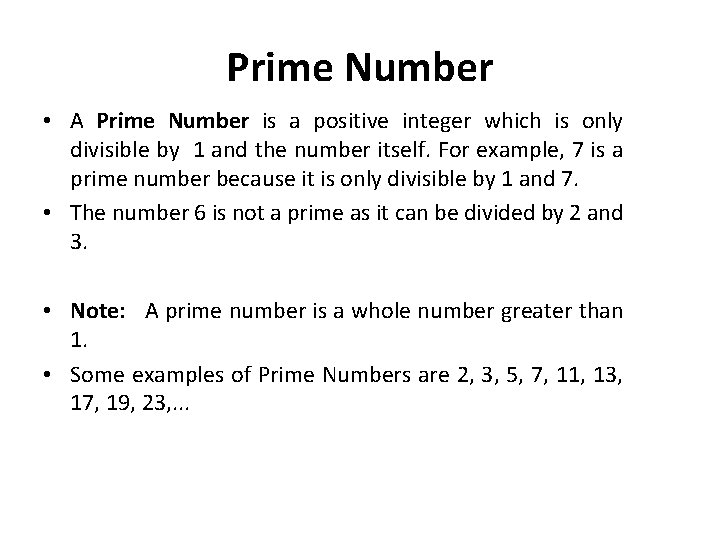Prime Number • A Prime Number is a positive integer which is only divisible by 1 and the number itself. For example, 7 is a prime number because it is only divisible by 1 and 7. • The number 6 is not a prime as it can be divided by 2 and 3. • Note: A prime number is a whole number greater than 1. • Some examples of Prime Numbers are 2, 3, 5, 7, 11, 13, 17, 19, 23, . . .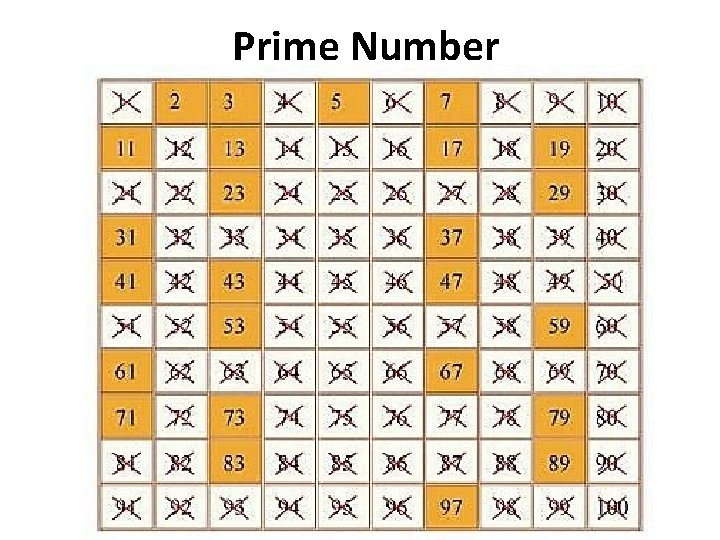Prime Number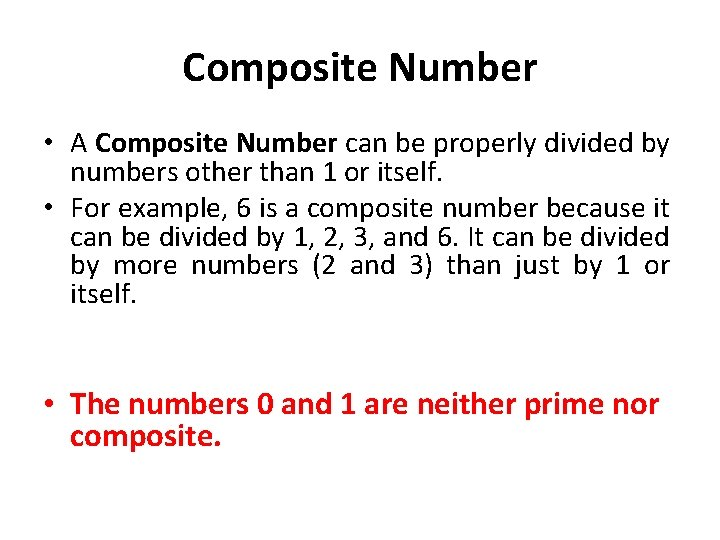Composite Number • A Composite Number can be properly divided by numbers other than 1 or itself. • For example, 6 is a composite number because it can be divided by 1, 2, 3, and 6. It can be divided by more numbers (2 and 3) than just by 1 or itself. • The numbers 0 and 1 are neither prime nor composite.Example • Determine whether each number is a prime or Composite numbers: • 0, 2, 4, 11, 37, 78, 79, 90, 1. • Prime numbers: • Composite numbers: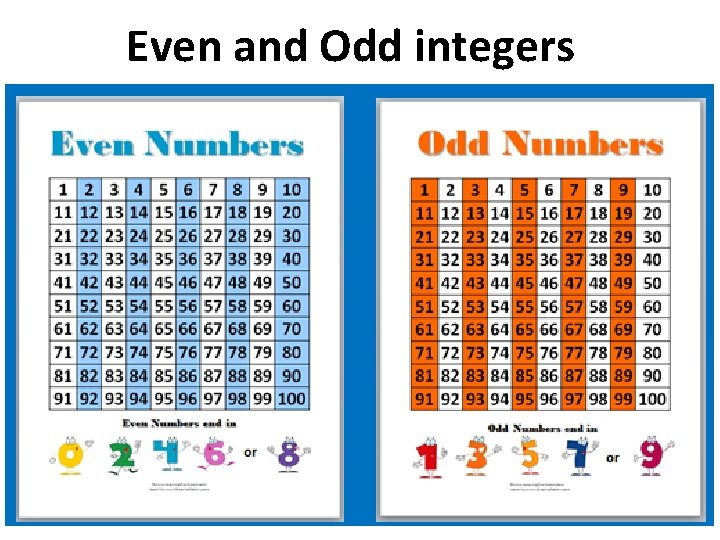Even and Odd integers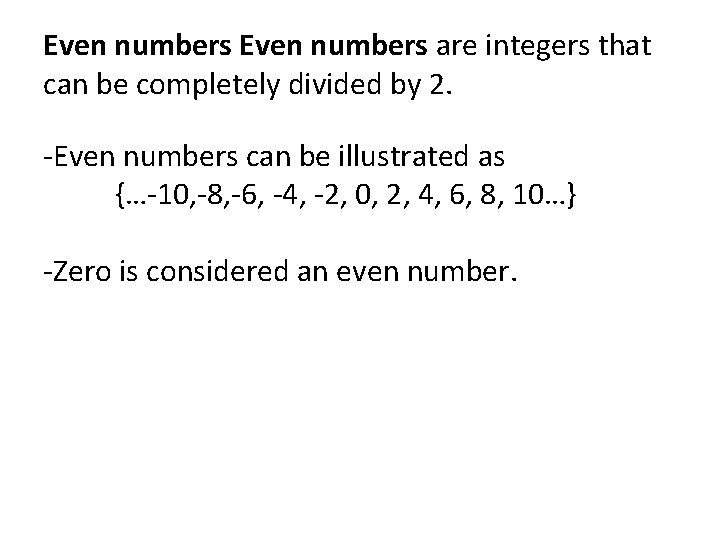Even numbers are integers that can be completely divided by 2. -Even numbers can be illustrated as {…-10, -8, -6, -4, -2, 0, 2, 4, 6, 8, 10…} -Zero is considered an even number.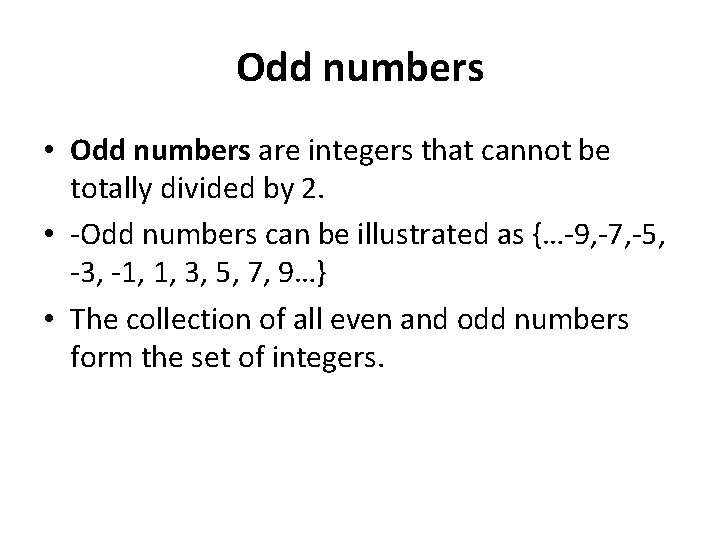Odd numbers • Odd numbers are integers that cannot be totally divided by 2. • -Odd numbers can be illustrated as {…-9, -7, -5, -3, -1, 1, 3, 5, 7, 9…} • The collection of all even and odd numbers form the set of integers.Note: • To determine whether a number is even or odd, notice the number at the ones place. Like to check if the numbers 12, 15, 36, 43, 69, 88, 101, 204 are even or odd, check the ones digit. • An even number ends in 0, 2, 4, 6, or 8. • An odd number ends in 1, 3, 5, 7, or 9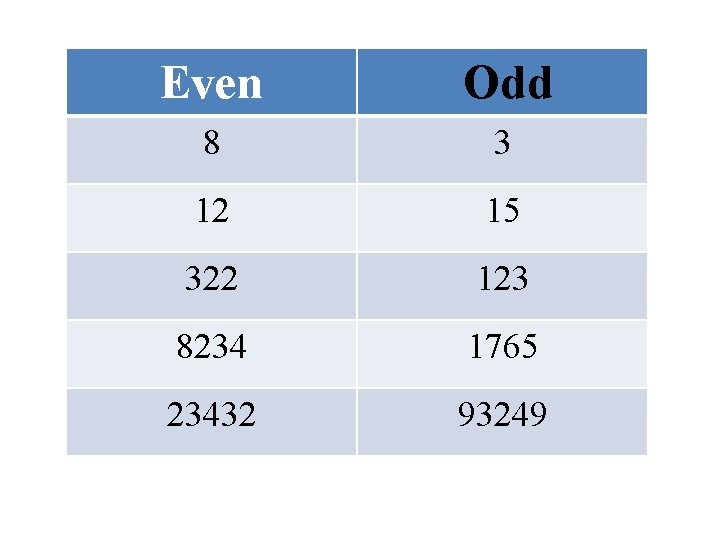Even Odd 8 3 12 15 322 123 8234 1765 23432 93249Rules of ASM Adding Evens and Odds even + even = even + odd = odd + odd = even Subtracting Evens and Odds even - even = even - odd = odd - odd = even Multiplying Evens and Odds even x even = even x odd = even odd x odd = odd 2 + 4 = 6 4 + 1 = 5 3 + 3 = 6 8 – 6 = 2 10 – 9 = 1 5 – 3 = 2 4 x 2 = 8 5 x 2 = 10 5 x 5 = 25Real Number System • Real Number System: The collection of all rational and irrational numbers form the set of real numbers, usually denoted by R.• Activity